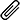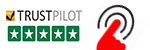High Quality, Fast Delivery, Plagiarism Free - Just in 3 Steps

## Upload Questions Details and Instructions:Select Assignment Files

## Get Assignment Help by Experts

### Plagiarism-Free, Every Time

All of our assignment solution delivers with a dedicated plagiarism report

### Excellent Subject Matter Expert

Get Supreme Assignment Help by highly Skilled Writers

### 100% Satisfaction Guaranteed

Score Higher or Your Money Back# (Get Answers) CPT_S 515 Midterm Exam: Do in Python Using pyEDA Package

i have a programming assignment which has to be solve using pyhton. CPT_S 515 Midterm Exam questions with answers in Python Using pyEDA Package Assessment. Python EDA Documentation PyEDA is a Python library for electronic design automation. Get case study answers to questions in Python Using pyEDA from programming experts online in Australia, UK and USA.i have a programming assignment which has to be solve using pyhton

## CPTS 515 Midterm Exam

Do in Python using pyEDA package. You may find installation instructions at https://pyeda.readthedocs.io/en/latest/install.html understand how CTL formulas are interpreted on a graph.

0. Let be a graph over 32 nodes (namely, node 0, · ·, node 31). For all 0 ≤ i, j  ≤31, there is an edge from node to node j  if· f (+ 3)%32 = j%32 or (+ 8)%32 = j%32. (% is the modular operator in C; e.g., 35% 32=3.) A node is even if is an even number. A node is prime if is a prime number.

In particular, we define [even] as the set {0, 2, 4 ·,· .30} and [prime] as the set {35711131719232931}. We use to denote the set of all edges in G.

1. (20%, graded on correctness and efficiency) (write-up) Let be a CTL formula while [p] is the set of nodes in that satisfy p.
• Please design a fix-point iteration algorithm that computes [EF p] (the set of nodes in that satisfy EF p from and [p]).
• Please design a fix-point iteration algorithm that computes [EGp] (the set of nodes in that satisfy EGp from and [p]).

You may first study the algorithms for [AGp] and [AF p].

1. (50%, graded on correctness and clarity. If you use explicit graph search such as DFS, you receive 0.) (Coding in Python) Every finite set can be coded as a BDD. Please write a Python program to compute [EG(even∧EF prime)] (the set of nodes that satisfy the formula EG( even∧ EF prime).), and verify your answer by checking node 5 satisfies EG(even∧ EF prime) and node 6 doesn’t satisfy EG(even∧ EF prime).

(Important: your code shall first encode R, [even], [prime] in BDDs using pyEDA and then using methods provided with the package, implement the iteration algorithms provided in 3 symbolically in BDD using methods in the package. Many students find methods BDD. Compose () and BDD. Smoothing () are quite useful in the package.)

1. (30%, graded on originality, depth, and quality of writing.) (write-up) To make use of the idea of BDDs, a major difficulty is which graph is chosen to model a given set of data. When the graph is too large, the symbolic exploration algorithm would not even run efficiently. However, if the graph is too small, the modeling might not be even realistic. We now think about an example problem. Consider a finite set of DNA strands, where each strand is simply a string on alphabet {A, T, C, G} (that is, a strand is a sequence of nucleotides, each of which is chosen from four nitrogen-containing nucleobases.). One may use a deterministic finite automaton (DFA)1

(which itself is a graph) to model the set L; i.e. L ⊆   L().

That is, each Strand in L, there is a walk-in (from the initial state to an accepting state) such that the symbols sequentially collected on the transitions on the walk form exactly the w. Clearly, many different can be used to model (e.g., can be ridiculously simple with only one state and accepting every word on the alphabet.). Consequently, one need come up with a metric Q(M, L), which is a real number, to characterize the “precision” on using the given to model L. When this is done, one can argue, for two given DFAs M1 and M2 which both model the given L, which model is more precise. Now, you need write a mini-paper (of 1 or 2 or more pages) in figuring out ways to define Q(M, L) and to design algorithms to compute Q(M, L).

#### Get This Answer for Study Help

If you need study assistance with writing your questions and answers, our professional assignment writing service is here to help!

#### Content Removal Request

If you are the original writer or copyright-authorized owner of this article and no longer wish to have, your work published on casestudyhelp.com, then please Request for removal of this content.

Top